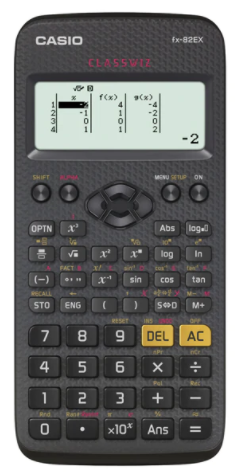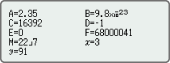# Casio FX-82EX Non Programmable Scientific Calculator #C71*Image may differ with actual product's layout, color, size & dimension. No claim will be accepted for image mismatch.

# Casio FX-82EX Non Programmable Scientific Calculator #C71

Product Id: 130.01.685.47

Regular Price  1,010
Special Price  950

Availability?

Quick Overview

Model - Casio FX-82EX
Brand - Casio
Type - Scientific Calculator
Display - Dot matrix display
Digit - 12 digits (10 + 2 digits)
Key - Plastic keys
Model
Casio FX-82EX
Type
Scientific Calculator
Display
Dot Matrix Display
Memory
No
Digit
12 (10 + 2 Digits)
Key
Plastic keys
Number of Functions
274
TAX Calculation
No
Power Sources
1 x AAA Battery
Specialty
Non Programmable Scientific Calculator, Natural textbook display, Display expression same as textbook, Intuitive, easy-to-learn icon display, The use of icons on the menu screen improves viewability, Selection of the desired function is quick and easy, Input and display fractions, powers, logarithms, roots, and other mathematical formulas and symbols just as they appear in textbooks, List display function for thorough, speedy learning, Easy-to-understand English messages and interactive format, A comprehensive set of advanced functions unique to CASIO scientific calculators, Multiline display, Variables list, Statistics list, Digit separator, 10 + 2 digits, Dot matrix display, Multi-replay, Plastic keys, Basic Mathematical Functions, Table Generation, In addition, graph functions can be represented by graphs, Intermediate Statistics, List based STAT-data editor, AAA-size Battery, Non Graphing, Basic Statistics
Others
Part No: C71, Natural textbook display: Display expression same as textbook, 10 + 2 digits: 10-digit mantissa + 2-digit exponential display, Dot matrix display: High-resolution screen provides beautiful looking graphs every time, Multi-replay: Quick and easy recall of previously executed formulas for editing and re-execution, Plastic keys: Designed and engineered for easy operation, Basic Mathematical Functions: Trigonometric, Exponential logarithmic, etc., Table Generation: can create a numerical table based on that expression by registering a function expression, Intermediate Statistics: More statistical functions such as Paired-variable statistical calculation, Quartiles and List display functions for speedy and thorough learning, List based STAT-data editor: Viewing and editing of input data in list format, showing data groups (x-data, y-data, frequency) and surrounding data, Basic Statistics: Standard statistics functions such as Mean, SUM, Standard Deviation, and Regression
Warranty
3 year
Made in/ Assemble
Thailand
Country Of Origin
Japan

## fx-82EX### Intuitive, easy-to-learn icon displayThe use of icons on the menu screen improves viewability. Selection of the desired function is quick and easy.

### Natural Textbook DisplayInput and display fractions, powers, logarithms, roots, and other mathematical formulas and symbols just as they appear in textbooks.

### List display function for thorough, speedy learningVariables and statistical calculation results stored in memory can be displayed in lists. There’s no need to recall and confirm individual values as with previous models.

### Easy-to-understand English messages and interactive formatNearly all English words are displayed on the screen in unabbreviated form. Interactive menu display realizes more intuitive operation.

### A comprehensive set of advanced functions unique to CASIO scientific calculators

Multiline displayFormulas are automatically wrapped to multiple lines. *

*In linear input mode

Variables listOne-touch list display

Statistics listDisplays six rows at once.

Digit separatorSeparation every three digits makes even large numbers easy to read.

Brand - Casio, Model - Casio FX-82EX, Type - Scientific Calculator, Digit - 12 (10 + 2 Digits), Display - Dot Matrix Display, Key - Plastic keys, Number of Functions - 274, TAX Calculation - No, Memory - no, Power source - 1 x AAA Battery, Others - Part No: C71, Natural textbook display: Display expression same as textbook, 10 + 2 digits: 10-digit mantissa + 2-digit exponential display, Dot matrix display: High-resolution screen provides beautiful looking graphs every time, Multi-replay: Quick and easy recall of previously executed formulas for editing and re-execution, Plastic keys: Designed and engineered for easy operation, Basic Mathematical Functions: Trigonometric, Exponential logarithmic, etc., Table Generation: can create a numerical table based on that expression by registering a function expression, Intermediate Statistics: More statistical functions such as Paired-variable statistical calculation, Quartiles and List display functions for speedy and thorough learning, List based STAT-data editor: Viewing and editing of input data in list format, showing data groups (x-data, y-data, frequency) and surrounding data, Basic Statistics: Standard statistics functions such as Mean, SUM, Standard Deviation, and Regression, Specialty - Non Programmable Scientific Calculator, Natural textbook display, Display expression same as textbook, Intuitive, easy-to-learn icon display, The use of icons on the menu screen improves viewability, Selection of the desired function is quick and easy, Input and display fractions, powers, logarithms, roots, and other mathematical formulas and symbols just as they appear in textbooks, List display function for thorough, speedy learning, Easy-to-understand English messages and interactive format, A comprehensive set of advanced functions unique to CASIO scientific calculators, Multiline display, Variables list, Statistics list, Digit separator, 10 + 2 digits, Dot matrix display, Multi-replay, Plastic keys, Basic Mathematical Functions, Table Generation, In addition, graph functions can be represented by graphs, Intermediate Statistics, List based STAT-data editor, AAA-size Battery, Non Graphing, Basic Statistics, Warranty - 3 year, Country of Origin - Japan, Made in/ Assemble - Thailand

## Your review## Your Question